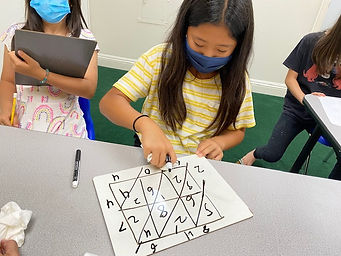## Ms. Dawn

### Target 1​

###### Lesson Type:

Continuation

Number Operation

:

Computation

Multiply within 1,000.

###### 1:

Understand there are many ways to multiply multi-digit numbers.

###### 2:

Understand the role of place-value in multiplication strategies.

###### 3:

Use the standard algorithm for solving multi-digit multiplication equations.

4th

###### Vocabulary:

Multiply, Product, Multiplication, Standard Algorithm, Lattice Method, Partial Product Method, Multi-digit

Activities:

1. Students used "Lattice Method" as a way solve a multiplication problem. Students solved 2-digit by 2-digit equations and also even solved 3-digit by 2-digit equations.

2. Students used their whiteboards to show they completely understand the "Lattice Method." They needed to draw out their own boxes to represent the equation they were solving.

3. Students solved a variety of multiplication equations using the "Partial Products Method." They needed to break it down using place value. They showed their understanding of what each digit was worth and why they had to multiply the numbers that way.

4. Students played a "Multiplication Fruit Punch" game. They rolled a die and moved that amount of spaces. If they landed on an equation, they had to solve it correctly in order to stay on that space. If they got it incorrect, they would move back to their previous space. If they landed on the "Free Space," they didn't need to solve any problem. If they landed on the "Fruit" pictures, they could move 3 spaces up. The first player to the finish line wins. We didn't get to finish the game.### Home Exploration

###### Guiding Questions:## Absent Students:

### Target 2

:

###### Vocabulary:

Activities:### Home Exploration

###### Guiding Questions:### Target 3

:

###### Vocabulary:

Activities:### Home Exploration